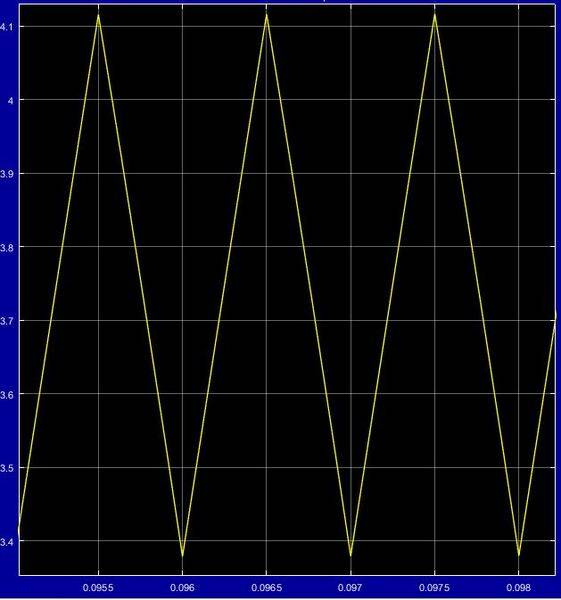# Boost converter in Simulink problem

Hi

I am trying to simulate a boost converter in Simulink, but I have a problem. When I measure the inductor current it shows as a triangular waveform instead of an exponential waveform like I would expect, does anyone know why? Does simulink do some simplification I am not aware of?

Parameters:
Inductor with parasitic resistance: 6.5e-3H, 0.01Ohm
Diode: 0.0001 Ohm on resistance and 0.8 forward voltage
Mosfet: 0.1 Ohm on resistance
Capacitor: 1000e-6 F

Simulation model:Inductor current:That's what inductor current normally looks like in a converter. You get a triangular ripple with an average centered about output current. If you were to drastically slow the switching frequency to where the converter was no longer operating properly you would see the hyperbolic part of the curve, but the on/off time is normally short enough to stay in the mostly linear region. You'd see the same thing with a capacitor if you were to charge and discharge with a short interval.

•OliskaP and berkeman
Aha, thank you.

Welcome, that ripple is a major point of design when it comes to inductor selection. Higher inductance values and higher switching frequencies result in less inductor current ripple. There are tradeoffs as always so you have to balance ripple with optimal efficiency, power requirements, and noise tolerance. Then there's the threshold for CCM/DCM. (continuous and discontinuous conduction modes). Inductance value and switching frequency affect that as well. The main thing is that output is typically noisier in DCM so in some cases you want to stay in CCM over a wider range of power outputs.

•OliskaP